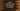# Swift program to find the first index of a character in a string## Swift program to find the first index of a character in a string:

In this swift tutorial, we will learn how to find the first index of a specific character in a string. Swift provides a lot of different utility methods for string and it makes it easier to do such types of string operations.

### Using firstIndex(of:)

firstIndex(of:) method can be used to find the first index of a character in a string. Below is the definition of this method :

``func firstIndex(of element: Character) -> Index?``

It takes one Character i.e. element as the parameter and returns the Index if found.

### Example of firstIndex(of:)

Let’s take a look at the below example:

``````let givenString = "Hello World !!"

let inputChar: Character = "o"

if let index = givenString.firstIndex(of: inputChar){
print(givenString[index])
}else{
}``````

Here, we are finding the index of character o in the string givenString. It will print the below output:

``o``

If you give any other character which is not available in the string, it will print Index not found !!.

### Using firstIndex(where:)

firstIndex(where:) is defined as below:

``func firstIndex(where: (Character) -> Bool) -> Index?``

It takes one predicate and based on that it returns the index if found. Else, it returns nil. This method is useful if we want to check if it satisfies a predicate not a specific character.

Let’s change the above program with this method:

``````let givenString = "Hello World !!"

let inputChar: Character = "o"

if let index = givenString.firstIndex(where: {\$0 == inputChar}){
print(givenString[index])
}else{